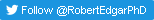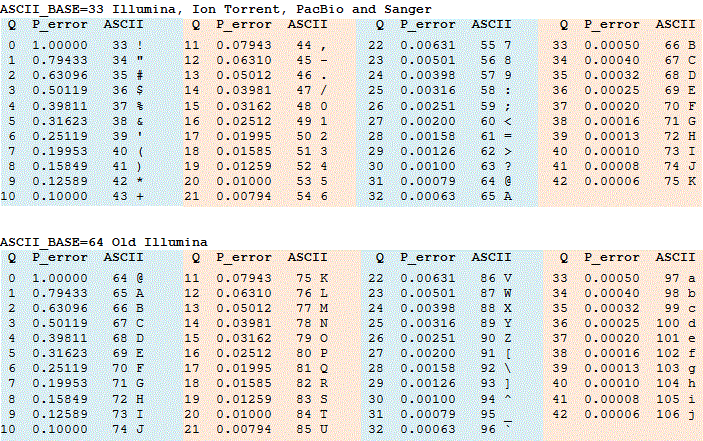﻿ Quality (Phred) scores11-Aug-2018 New paper describes octave plots for visualizing alpha diversity.

12-Jun-2018 New paper shows that one in five taxonomy annotations in SILVA and Greengenes are wrong.

18-Apr-2018 New paper shows that taxonomy prediction accuracy is <50% for V4 sequences.

05-Oct-2017 PeerJ paper shows low accuracy of closed- and open-ref. QIIME OTUs.

22-Sep-2017 New paper shows 97% threshold is wrong, OTUs should be 99% full-length 16S, 100% for V4.

24-Nov-2016Quality (Phred) scores

The quality score of a base, also known as a Phred or Q score, is an integer value representing the estimated probability of an error, i.e. that the base is incorrect. If P is the error probability, then:

P = 10-Q/10

Q = -10 log10(P)

Q scores are often represented as ASCII characters. The rule for converting an ASCII character to an integer varies, see FASTQ options for details. Tables converting between integer Q scores, ASCII characters and error probabilities are shown in the table below ASCII_BASE 33, which is now almost universally used, and ASCII_BASE 64 which is used in some older Illumina data.What kind of error?
There is an important difference between Q scores in reads from 454 and Illumina. In effect, 454 ignores the possibility of substitution errors and Illumina ignores indels. With 454, the Q score is the estimated probability that the length of the homopolymer is wrong, and with Illumina the Q score is the probability that the base call is incorrect. In the case of Illumina, this is reasonable because indel errors are very rare. But with 454, substitution errors are quite common, occurring with comparable frequency to homopolymer errors. This means that 454 Q scores are not as informative as Illumina Q scores, but are still useful in practice. See quality filtering for further discussion.

Small Q scores
Note that a Q score of 3 means P=0.5, meaning that there is a 50% chance the base is wrong, and lower values represent even higher probabilities of error. Q=0 means P=1, i.e. that the base call is certainly wrong, so this is rarely used, though might be appropriate for an undetermined base (often represented as 'N'). I have never seen a FASTQ file with Q=0, but since the format is not standardized I can't be sure. The lowest value usually found in practice is Q=2 (P=0.63), which means the base call is more likely to be wrong than correct.

Recognizing the format
The fastx_info and fastq_chars commands can be used to determin the format. The most important parameter is ASCII_BASE, which as far as I know is always 33 or 64. With a typical range from Q2 to Q40, this gives a range of ASCII values from 35 to 73 with ASCII_BASE=33 and from 66 to 104 with ASCII_BASE=64. These ranges overlap from ASCII 66 to 73. Also, values >Q40 may be produced by some machine software and by some post-processing software such as paired read assemblers. So if we see ASCII values >73 that doesn't necessarily mean that we have ASCII_BASE=64, these could be high quality scores with ASCII_BASE=33. The only sure way to distinguish for sure is if we see ASCII values < 64, in which case we know ASCII_BASE=33. A quick way to check visually is to look for # and \$, which means ASCII_BASE=33 or lower-case letters which probably implies ASCII_BASE=64.